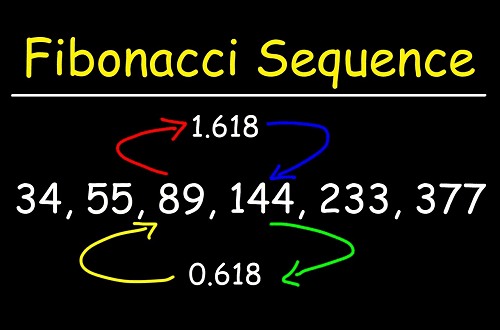# Fibonacci Series implementation in C programming

## Fibonacci implementation

The Fibonacci Sequence is the series of numbers: 0, 1, 1, 2, 3, 5, 8, 13, 21, 34, ... The next number is found by adding up the two numbers before it. The 2 is found by adding the two numbers before it (1+1). Similarly, the 3 is found by adding the two numbers before it (1+2), And the 5 is (2+3), and so on! The first two numbers in the Fibonacci sequence are either 1 and 1 or 0 and 1, depending on the chosen starting point of the sequence, and each subsequent number is the sum of the previous two. It is an interesting series, and we all know it very well. But how many of us use it in our practical life??? Now try to solve a problem by implementing the Fibonacci series.Implementing Fibonacci

Let's see some easy ACM problems.

#### The Fibonacci Series implementation in C programming:

#include<stdio.h>

int main() {
int n1 = 0, n2 = 1, n3, i, number;
printf("Enter the number of elements:");
scanf("%d", & number);
printf("\n%d %d", n1, n2); //printing 0 and 1
for (i = 2; i < number; ++i) //loop starts from 2 because 0 and 1 are already printed
{
n3 = n1 + n2;
printf(" %d", n3);
n1 = n2;
n2 = n3;
}
return 0;
}code-box